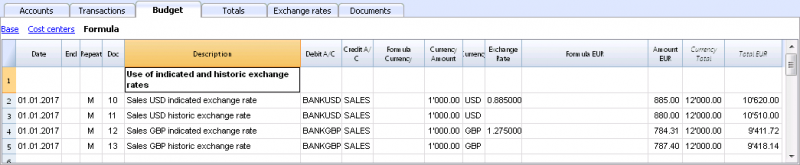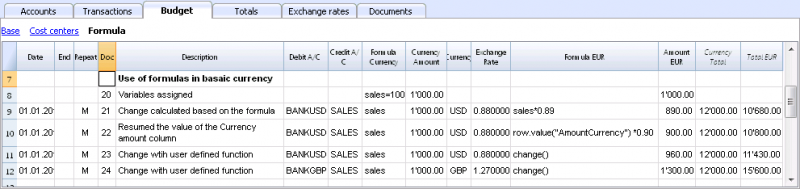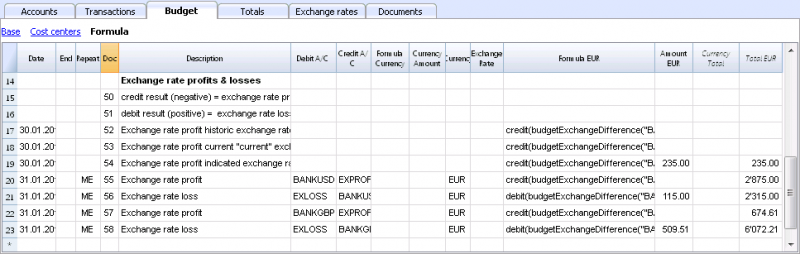Multi-Currency Financial Planning with formulas

Luke's coffeehouse

Basic Currency: EUR

Double-entry with foreign currencies

Accounts
Group Account Description Currency Gr
BALANCE SHEET
ASSETS
CASH Cash on hand EUR 1
BANK Bank account EUR 1
BANKUSD USD Bank account USD 1
BANKGBP GBP Bank account GBP 1
CUSTOMERS Customers account EUR 1
FURNITURE Furniture EUR 1
INVENTORY Inventory EUR 1
1   TOTAL ASSETS   00

LIABILITIES AND EQUITY
SUPPLIERS Suppliers EUR 2
LOAN Loan EUR 2
EQUITY Owner equity EUR 2
PL   Profit(-) or loss (+) from current year   2
2   TOTAL LIABILITIES AND EQUITY   00

PROFIT AND LOSS STATEMENT
INCOME
SALES Income from sales EUR 4
EXPROFIT Exchange rate profit EUR 4
INTINC Interest income EUR 4
4   TOTAL INCOME   02

EXPENSES
PERSON Personnel expenses EUR 3
GOODS Cost of goods EUR 3
RENTAL Rental and related expenses EUR 3
INTEXP Interest expense EUR 3
EXLOSS Exchange rate loss EUR 3
DEPREC Depreciation EUR 3
3   TOTAL EXPENSES   02

02   Profit(-) Loss(+) from Profit and Loss Statement   PL

00   Difference should be zero (void)

Exchange rates
Ref.Currency Currency Text
USD US Dollars
GBP British Pounds
USD US Dollars
USD US Dollars
USD US Dollars
USD US Dollars
USD US Dollars
USD US Dollars
USD US Dollars
USD US Dollars

GBP British Pounds
GBP British Pounds
GBP British Pounds
GBP British Pounds
GBP British Pounds
GBP British Pounds
GBP British Pounds
GBP British Pounds

This example will explain how to use formulas specific to double-entry bookkeeping with multi-currency functionalities.

Banana Accounting version 8.0.5 is needed to use these functions.

In previous versions certain functions are not available and error message will show.

Please refer to the following pages for further information:

Documentation on use of formula in the Budget table.

Documentation on use of formula in the Budget table.

Amount calculation in base currency

The formula will calculate the value in the account's currency first and then the relative value in the base currency.

Exchange rates and historical rates• Doc 10 and 12.
If an exchange rate is indicated in the exchange rate column, the program will calculate the amount in the base currency (EUR in this case) using the exchange rate indicated in this row.
• Doc 11 and 13.

If there is no exchange rate indicated, the program will apply the exchange rate in the Exchange rates table for this calculation as well as for the successive operation in the case a Repeat function is applied.

• If historical exchange rates are defined in the Exchange rates table, via adding a date, the program will apply the exchange relative to the date or the previous, most recent one.

Use of formulas in the base currencyAll formulas available in the Budget table can be used in the Formula column.

The following examples show the value in the base currency (EUR) calculated according to a Formula.

• Doc 21.
The sales value is multiplied according to the Formula EUR value. The exchange rate in the corresponding column will be ignored.
• Doc 22.
As in the previous example, but for the value of "AmountCurrency" which is the result of the formula.
• Doc 23.
In the “budget.js” document we have defined an exchange rate function calculating the value on the basis of the contents in the row.
With these functions it is possible to create exchange rate simulations.

Example for Exchange rate function

The USD exchange rate is established on a monthly basis.

A constant exchange rate is used for GBP instead.

The function will result as the amount of AmountCurrency multiplied by the exchange rate.

function cambio() {
var importo = row.value("AmountCurrency");
var cambio = 1;
var moneta = row.value("ExchangeCurrency");
var data = Banana.Converter.toDate(row.value("JDate"));
var mese = data.getMonth() + 1;
if (moneta == "USD") {
cambio = 0.95;
if (mese == 1)
cambio = 0.96;
else if (mese == 2)
cambio = 0.97;
}
if (moneta == "GBP") {
cambio = 1.30;
}
return importo * cambio;
}

Formulas for calculation of exchange rate differences

For further information on calculation of exchange rate differences please refer to informations about transactions in multi currency and to the Create exchange rate differences command.• The currency is always the base currency.
• Amounts must not be entered in the account's currency.
• Exchange rate is left blank and will be ignored, even if a value is entered.
• The budgetExchangeDifference function calculates the exchange rate difference for an account. This value represents the non realized exchange rate profit or loss.
.
• The function will return a positive value if it is an exchange rate loss and a negative (credit) in case of exchange rate profit.

Exchange rate profit and exchange rate loss need to be entered in two different rows.

• The resulting amount represents the revaluation necessary in the base currency to ensure that the account balance in the base currency matches the balance of the account in the currency converted at the exchange rate used for the calculation of exchange rate differences.

• The first parameter "CUSD" of the budgetExchangeDifferences function is the account in foreign currency for which the exchange rate difference will be calculated.
• The second parameter is the exchange rate to be applied.
• If no exchange rate is indicated, the historical exchange rate relative to the date of the entered transaction is applied.
• When "current" is used, the exchange in the Exchange rate tables with no date will be used.
• An exchange my also be indicated. A point "." must be used as a decimal separator.

credit(budgetExchangeDifference("CUSD"))
credit(budgetExchangeDifference("CUSD", "current"))
credit(budgetExchangeDifference("CUSD", "0.95"))